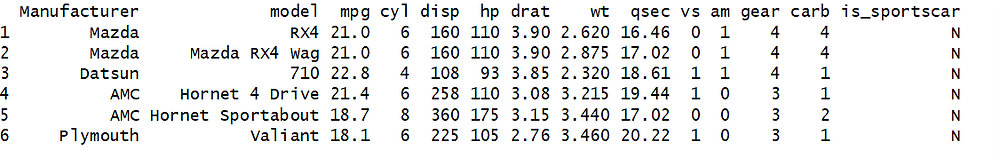Search

# Creating Bar Charts: Step Twelve in Learning R Programming for Free

I hope you are enjoying the “Learning R Programming for Free” series; here are links to the previous segments (Step OneStep TwoStep ThreeStep FourStep FiveStep SixStep SevenStep EightStep NineStep Ten, Step Eleven) to provide some helpful background.

In the previous installment, we discussed the functions in the “rio package.” In this segment, we extend our mtcars data set, and then use ggplot to create some bar charts.

Bar charts are very useful when we need to count things.  We can also use them to compare counts.

Let’s make sure rio is installed:

install.packages(“rio”)

library(rio)

The data set we will use was built in Step Eight of this blog series.  Let’s save our updated mtcars data set from Step Eight to an Excel file:

export(mydf, “c:/mycars.xlsx”)

and now if we go to our C: drive, we find our cars data in mycars.xlsx:Next, let’s add a manufacturer’s column and a Boolean metric indicating if the car is a sports car to our data set.  Then, we will re-import the enhanced data:

mycars <- import(“c:/mycars1.xlsx”)We can see that we now have two characters that look like an arrow, <- (this is one of the assignment operators in R).  In this case, we are loading (assigning) the contents of our Excel file into the object “mycars.”

The head function is handy to look at the first few lines of a data set. It takes an object as an argument and it functions on vector, matrix, table, data frame or function classes.

You may recall earlier in our installments when we used class() to get our object type?

class(mycars)

 “data.frame”

Our “mycars” data is of type “data.frame.”  This will be important later on when we learn certain functions only work on certain types of data.  We will continue that discussion later.  For now, remember that when we use the import function from the rio package, it creates data frames.  Now, back to ggplot.

Let’s take a look at ggplot bar charting features.  Here is a very simple plot of the count of cars with a certain number of cylinders, 4, 6 and 8:

ggplot(mycars, aes(cyl)) + geom_bar()Not the most beautiful chart ever made but it’s a place to start.  A few things to notice.  We only had to declare what we wanted to count, “cyl.”  For bar charts in ggplot, the count is calculated automatically through a built-in binning feature.

Let’s break this down a bit.  To use ggplot, we need three basic things: DATA, AESTHETICS, and GEOMETRY.  Ggplot allows us to layer multiple geometries.  We can also label our plot, add colors and themes.

First, we call ggplot. Then, the first argument is the data frame we want to use.  The second argument is what is called the aesthetics (aes).  Then we use the plus sign to append on the chart type we want.  For bar charts, that would be “geom_bar().”